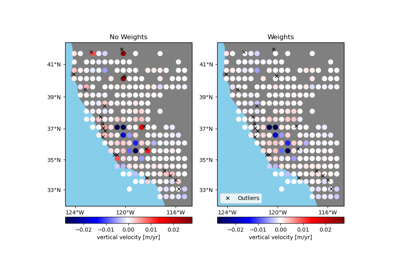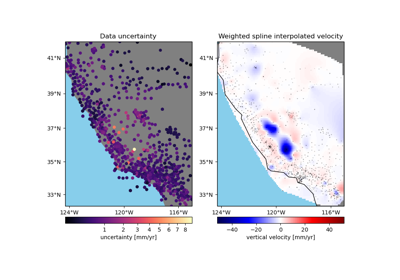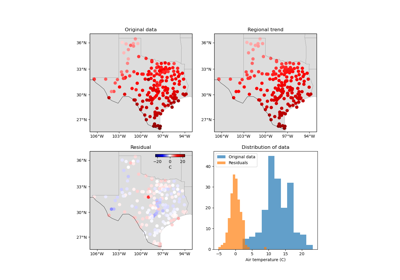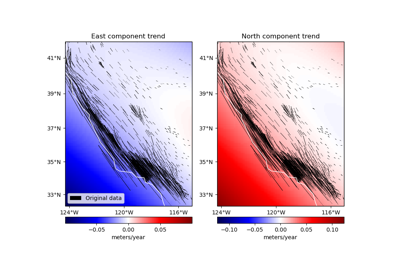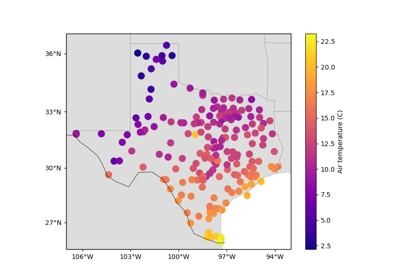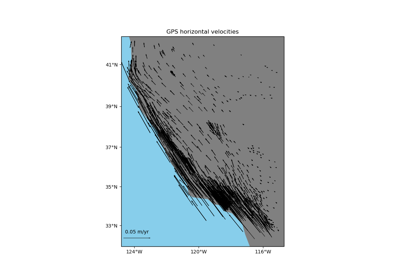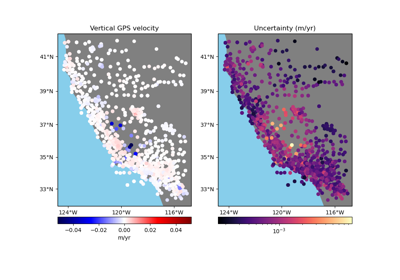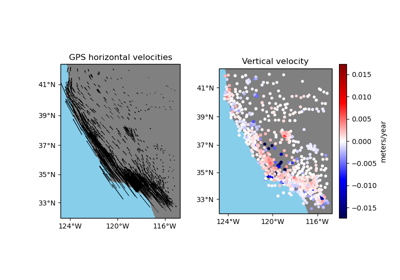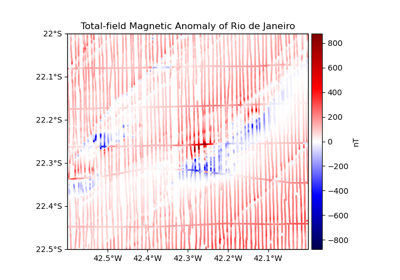# verde.maxabs¶

verde.maxabs(*args, nan=True)[source]

Calculate the maximum absolute value of the given array(s).

Use this to set the limits of your colorbars and center them on zero.

Parameters

args – One or more arrays. If more than one are given, a single maximum will be calculated across all arrays.

Returns

maxabs (float) – The maximum absolute value across all arrays.

Examples

>>> maxabs((1, -10, 25, 2, 3))
25
>>> maxabs((1, -10.5, 25, 2), (0.1, 100, -500), (-200, -300, -0.1, -499))
500.0


If the array contains NaNs, we’ll use the nan version of of the numpy functions by default. You can turn this off through the nan argument.

>>> import numpy as np
>>> maxabs((1, -10, 25, 2, 3, np.nan))
25.0
>>> maxabs((1, -10, 25, 2, 3, np.nan), nan=False)
nan


## Examples using verde.maxabs¶